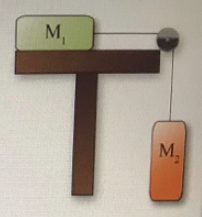# Problem: A block with mass m1 = 7.1 kg rests on the surface of a horizontal table which has a coefficient of kinetic friction of μ = 0.57. A second block with a mass m2 = 9.6 kg is connected to the first by an ideal pulley system such that the second block is hanging vertically. The second block is released and motion occurs.A. Using the variable T to represent tention, write an equation for the sum of the forces in the y-direction, for block two.B. Using the variable T to represent tension, write an expression for the sum of the forces in the x-direction for block 1.C. Write and expression for the magnitude of the acceleration of block 2, in terms of the acceleration of block one. (Assume the cable connecting the masses is ideal).D. Write an expression using the variable provided for the magnitude of the tension force T.E. What is the tension, T, in newtons?

###### FREE Expert Solution

Newton's second law:

$\overline{){\mathbf{\Sigma }}{\mathbf{F}}{\mathbf{=}}{\mathbf{m}}{\mathbf{a}}}$

A.

The motion of block 2 is contributed to by the sum of forces in the y-direction.

ΣFy = m2g - T

The equation for the sum of the forces in the y-direction, for block two is ΣFy = m2g-T.

B.

For block 1, the forces are in the x-direction.

97% (441 ratings)###### Problem Details

A block with mass m1 = 7.1 kg rests on the surface of a horizontal table which has a coefficient of kinetic friction of μ = 0.57. A second block with a mass m2 = 9.6 kg is connected to the first by an ideal pulley system such that the second block is hanging vertically. The second block is released and motion occurs.A. Using the variable T to represent tention, write an equation for the sum of the forces in the y-direction, for block two.

B. Using the variable T to represent tension, write an expression for the sum of the forces in the x-direction for block 1.

C. Write and expression for the magnitude of the acceleration of block 2, in terms of the acceleration of block one. (Assume the cable connecting the masses is ideal).

D. Write an expression using the variable provided for the magnitude of the tension force T.

E. What is the tension, T, in newtons?